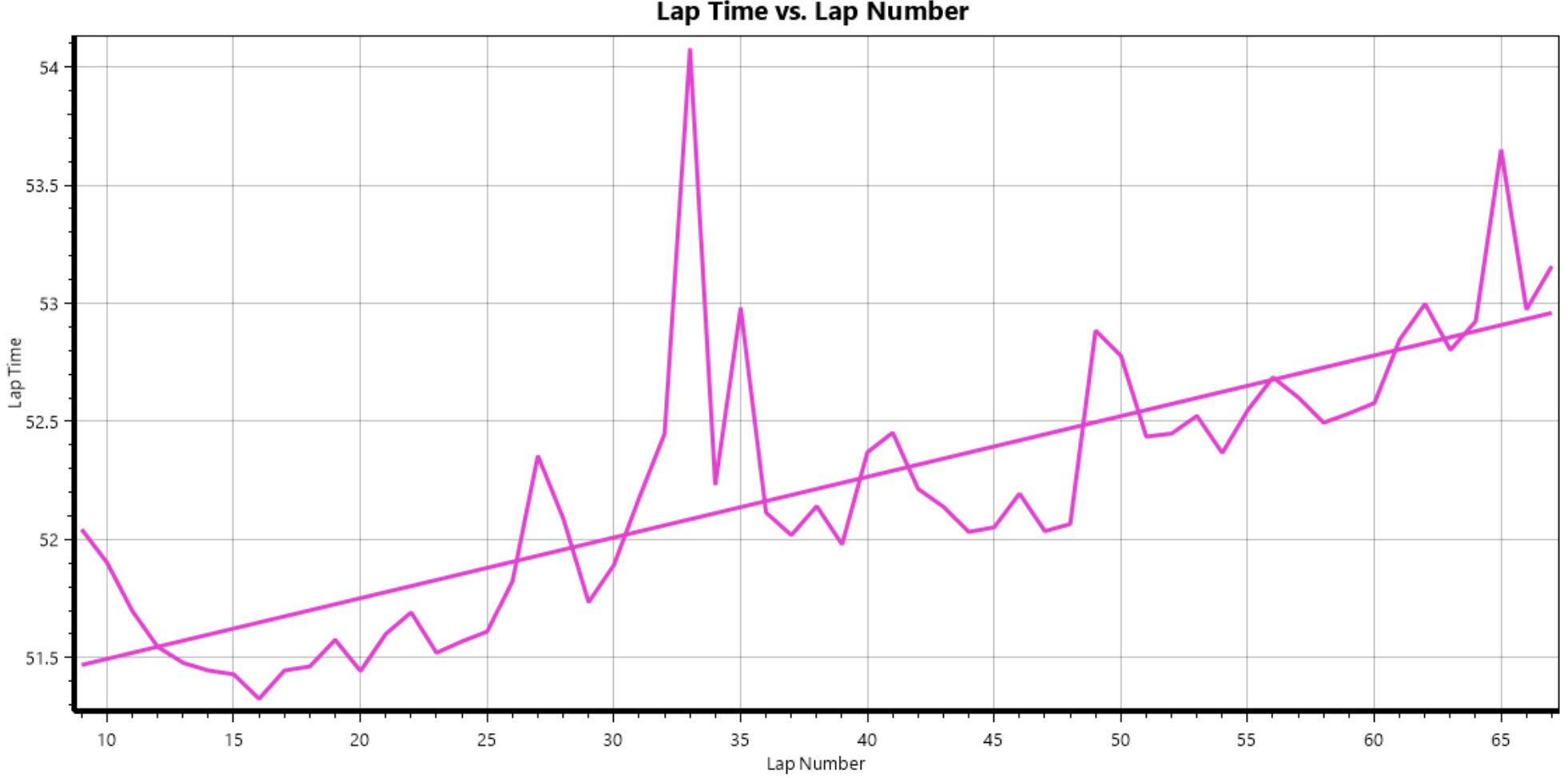The degradation calculation is completed for each stint (outing) for each car individually. The degradation number shown is the slope of the line fitted to all the green flag laps of each stint. An example of the linear fit for a stint is shown below.LapTime Degradation is the linear best fit of laptime vs LapNumber. The calculation does not take into account the following laps:

• InLap
• OutLap
• SC
• FCY
• Laps with a laptime greater than 120% of the average laptime in the current stint

This output can be displayed in a scatter plot, a strategy overview, and a Stint Analysis.